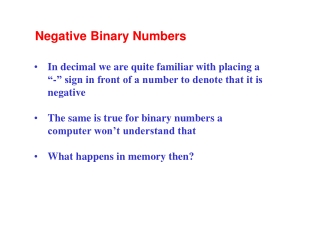DownloadDownload PresentationIn decimal we are quite familiar with placing a

# In decimal we are quite familiar with placing a

Download Presentation## In decimal we are quite familiar with placing a

- - - - - - - - - - - - - - - - - - - - - - - - - - - E N D - - - - - - - - - - - - - - - - - - - - - - - - - - -
##### Presentation Transcript

1. Negative Binary Numbers • In decimal we are quite familiar with placing a • “-” sign in front of a number to denote that it is negative • The same is true for binary numbers a computer won’t understand that • What happens in memory then?

2. Binary Negative Numbers • There are several representations • - Signed magnitude • - One’s complement • - Two’s complement • Two’s complement is the system used in microprocessors • Most significant bit becomes important

3. Signed Magnitude • Represent the decimal number as binary • Left bit (MSB) used as the sign bit • Only have 7 bits to express the number • 1210 = 00001100 • -1210 = 10001100 • How many representations are there for zero?

4. One’s Complement • Method: Invert the ones and zeros • 1110 = 00001011 • -1110 = 11110100 • 0 in MSB implies positive • 1 in MSB implies negative

5. Two’s Complement • Method: Take the one’s complement and add 1 • 1110 = 00001011 • -1110 = 11110100 one’s comp • -1110 = 11110101 two’s comp

6. Why Two’s Complement? • One representation of zero (Check) • Enables subtraction operation by considering the addition of a positive number with a two’s complement number • Only need addition • MSB indicates the sign of the number

7. One Representation of 0 • 00000000 • 11111111 • (1) 00000000

8. Subtraction by Addition • Consider 10 – 6 using binary subtraction • 00001010 10 • 00000110 6 • 00000100 subtract gives 4

9. Two’s complement • -6 • Binary conversion of 6 = 00000110 • One’s complement (invert) = 11111001 • Two’s complement (+1) = 11111010

10. Subtraction by Addition • Consider 10 – 6 using two’s complement • 0001010 10 • 11111010 6 • (1) 00000100 adding gives 4

11. . Why two’s complement • Only need one type of hardware/process to add both signed and unsigned numbers.